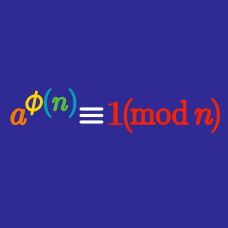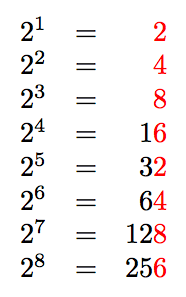Number Theory

# Euler's Theorem: Level 2 ChallengesWhat is the last digit of $$2^9?$$

For a positive integer $$n>2,$$ what can we say about the number of positive integers less than $$n$$ that are relatively prime to $$n$$ (that is, their GCD is 1)?

Hint: A useful property of the Greatest Common Divisor function is $$\gcd(a,b) = \gcd(a,a-b);$$ e.g., $$\gcd(10,8) = \gcd(10,2) = 2.$$

Find the last two digits of $$7^{100}-3^{100}.$$

What is the largest integer that always divides $$n^{7}-n$$ for any integer $$n?$$

$\LARGE \color{blue}5^{\color{green}5^{\color{red}5^{\color{brown}5^\color{magenta}5}}}$

What are the last three digits of the number above?

×

Problem Loading...

Note Loading...

Set Loading...